# Octagon

An octagon is a polygon that has eight sides. In the figure below are 3 different types of octagons. A stop sign is an example of objects in everyday life that is in the shape of an octagon.

## Octagon classifications

Like other polygons, an octagon can be classified as regular or irregular. If all the sides and interior angles of an octagon are equal, it is a regular octagon. Otherwise it is an irregular octagon.

Regular octagonIrregular octagon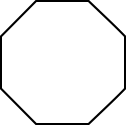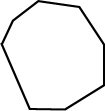All sides and interior angles are equal Not all sides and angles are equal

Octagons and other polygons can also be classified as either convex or concave. If all interior angles of an octagon or polygon are less than 180°, it is convex. If one or more interior angles are larger than 180°, it is concave. A regular octagon is always a convex octagon.

Convex octagonConcave octagon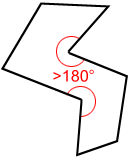All interior angles < 180° One or more interior angles > 180°

## Parts of an octagon

### Diagonals of an octagon

A diagonal is a line segment joining two non-consecutive vertices. Five diagonals can be drawn from each vertex. A total of 20 diagonals can be drawn for an octagon. The following figure is an example.

### Internal angles of an octagon

The sum of the interior angles of an octagon equals 1080°.

As shown in the figure above, five diagonals can be drawn to divide the octagon into six triangles. The blue lines above show just one way to divide the octagon into triangles; there are others. The sum of interior angles of the six triangles equals the sum of interior angles of the octagon. Since the sum of the interior angles of a triangle is 180°, the sum of the interior angles for the hexagon is 6 × 180° = 1080°.

## Regular octagons

A regular octagon is an octagon whose sides are equal in length, and whose interior angles are equal in measure.

• Since each of the eight interior angles in a regular octagon are equal in measure, each interior angle measures 1080°/8=135° as shown below.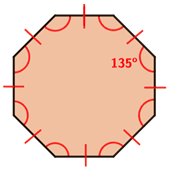• Each exterior angle for a regular octagon has an equal measure. The exterior angles of a regular octagon have an angle of 45°.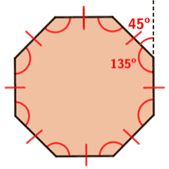• Sides of a regular octagon are equal in length, and the opposite sides of a regular octagon are parallel.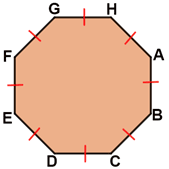AB=BC=CD=DE=EF=FG=GH=AH and AB//EF, BC//FG, //GH, DE//AH

### Symmetry in regular octagons

A regular octagon has 8 lines of symmetry and a rotational symmetry of order 8. This means that it can be rotated in such a way that it will look the same as the original shape 8 times in 360°.

Lines of symmetryRotational symmetry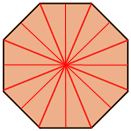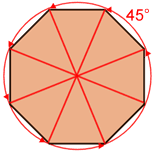8 lines of symmetry Eight 45°angles of rotation

### Area of a regular octagon

The area of a regular octagon with side length s is:Extending the 4 non-adjacent sides of a regular octagon with side length s will create a square as shown by the dashed blue lines above. It also forms four 45-45-90 right triangles on the four corners of the square. The hypotenuse of the right triangles are s. The leg lengths of the right triangles are. Accordingly, the side length of the square is. The area of the octagon can be calculated by subtracting the areas of the four right triangles from the area of the square.

 A ====Example:

What is the area of a regular octagon that has a perimeter of 40?

Since the perimeter is 40, we can find s as follows,

 P = 8 × s 40 = 8 × s s = 5

Using:

 A === 120.71